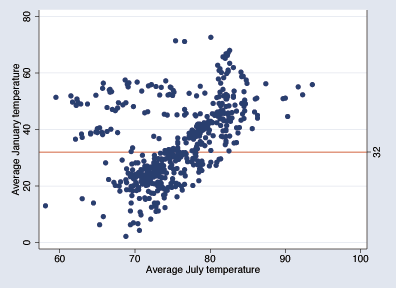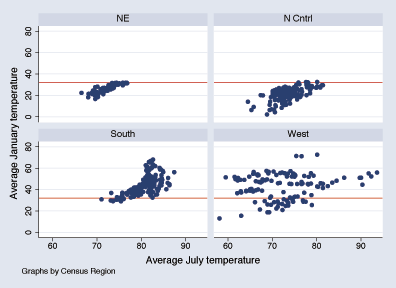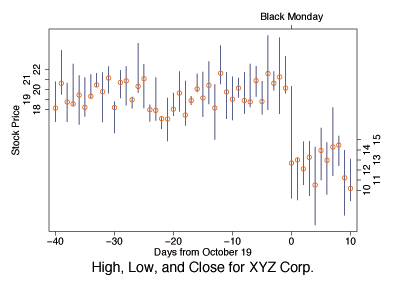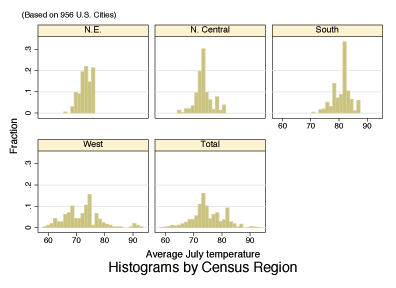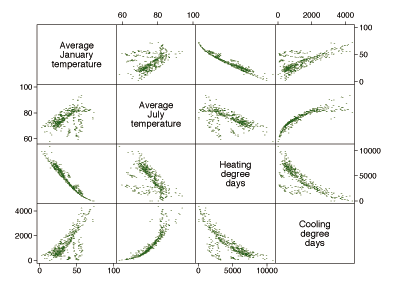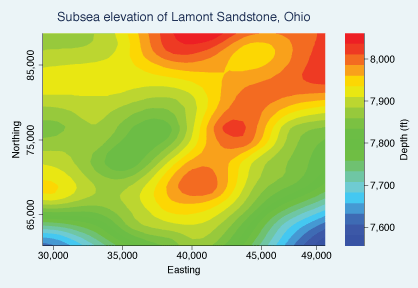»  Home »  Products »  Features »  Graphics »  Graph styles

## Graph styles

 Bar charts Box plot Histogram Spike plots Pie charts Scatterplot matrix Dot chart Line charts Area charts Two-way scatterplot Contour plots

The figure below was made using Stata.

Each of the basic styles corresponds to a style option:

• The histogram at the top left was made by typing histogram mpg.
• The two-way scatterplot in the second column of the first row was drawn by typing scatter price mpg.
• The scatterplot matrix in the third column of the first row was obtained by typing graph matrix price mpg weight length.
• The box-and-whisker plot in the upper-right corner was obtained by typing graph box weight, over(foreign, total).
• The bar chart was obtained by typing graph bar rep1-rep5.
• The horizontal-bar chart was obtained by typing graph hbar (mean) mpg, over(manuf).
• The dot chart was obtained by typing graph dot (mean) mpg, over(manuf).
• The fitted-values chart was obtained by using overlaid two-way graphs and typing scatter mpg weight || line lo weight || line hi weight || line hat weight.
• The line graph was obtained by typing line weight mpg, sort.
• The combined figure was obtained by (1) saving each of the graphs when we drew them and (2) typing graph combine followed by the names under which the images were saved.

An important feature of Stata is that it has no modes or modules. The graphics commands are always available, so you can fit a regression and graph the residuals without performing computer gymnastics. Stata's graphs are designed not only to look good but to be informative analytic tools.

Below are some images produced by Stata.